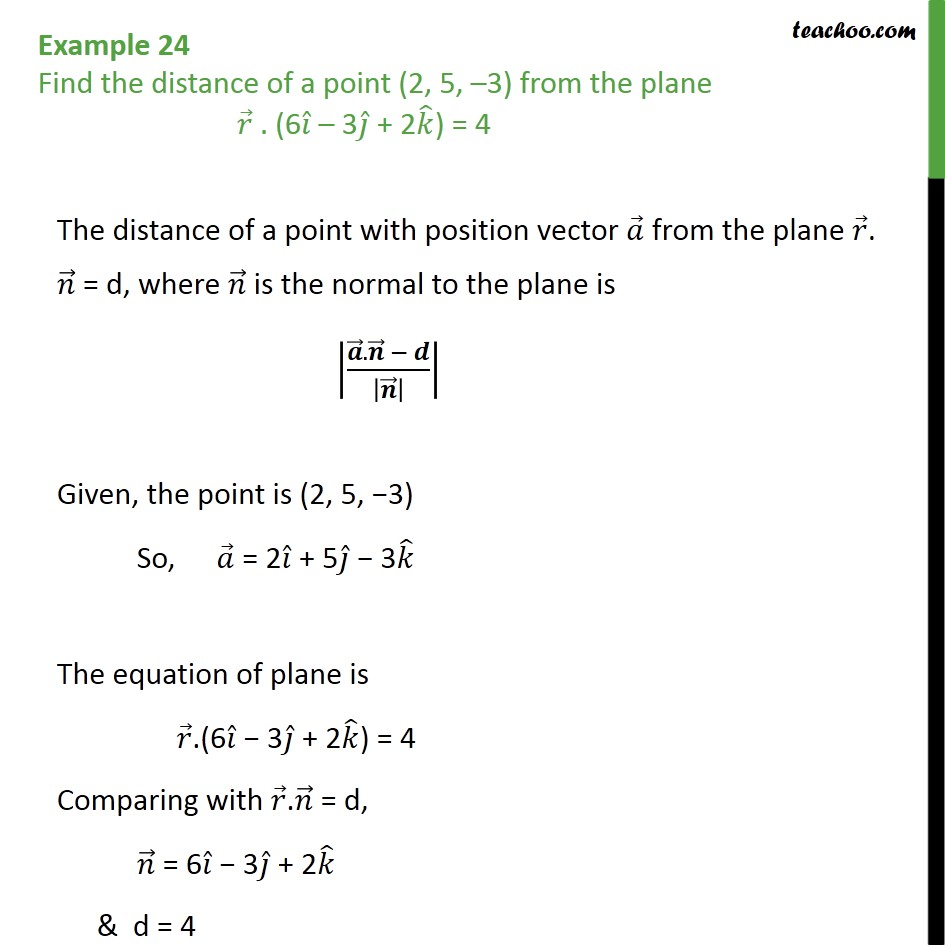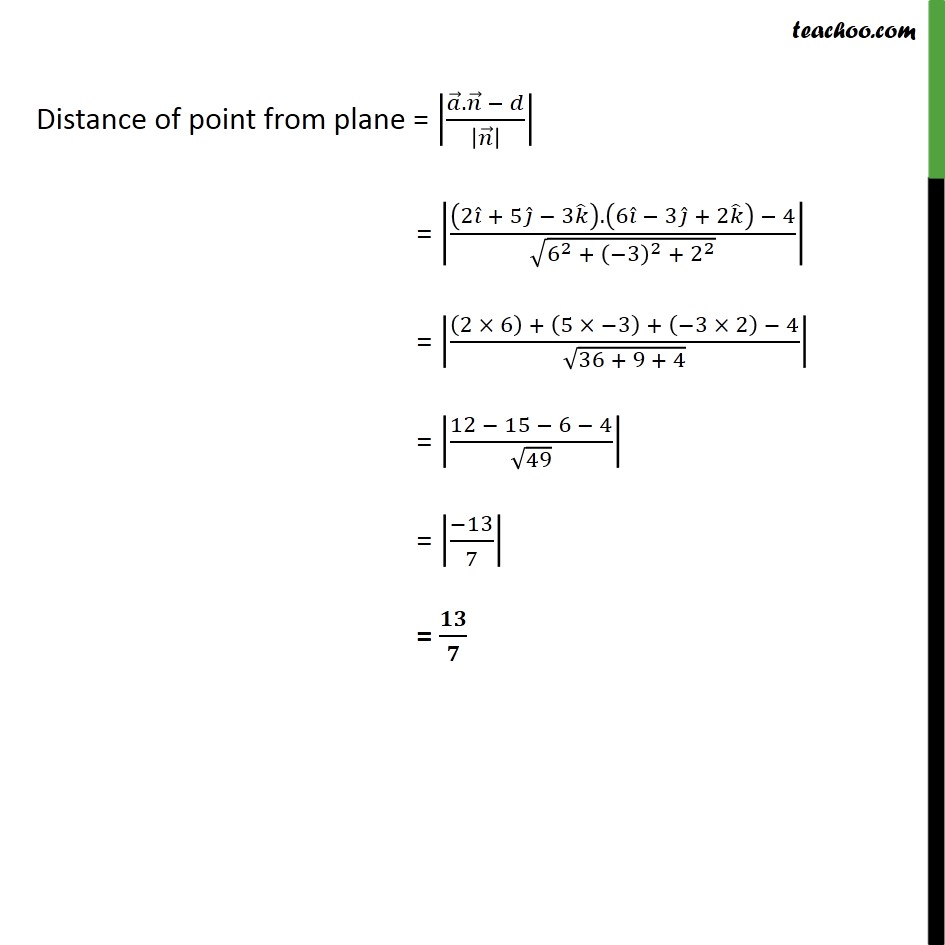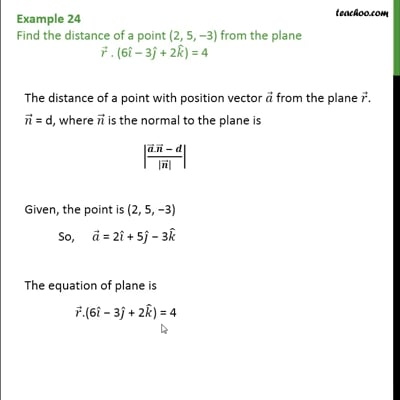Examples

Chapter 11 Class 12 Three Dimensional Geometry
Serial order wiseThis video is only available for Teachoo black users

Solve all your doubts with Teachoo Black (new monthly pack available now!)

### Transcript

Example 24 Find the distance of a point (2, 5, –3) from the plane 𝑟﷯ . (6 𝑖﷯ – 3 𝑗﷯ + 2 𝑘﷯) = 4 The distance of a point with position vector 𝑎﷯ from the plane 𝑟﷯. 𝑛﷯ = d, where 𝑛﷯ is the normal to the plane is 𝒂﷯. 𝒏﷯ − 𝒅﷮ 𝒏﷯﷯﷯﷯ Given, the point is (2, 5, −3) So, 𝑎﷯ = 2 𝑖﷯ + 5 𝑗﷯ − 3 𝑘﷯ The equation of plane is 𝑟﷯.(6 𝑖﷯ − 3 𝑗﷯ + 2 𝑘﷯) = 4 Comparing with 𝑟﷯. 𝑛﷯ = d, 𝑛﷯ = 6 𝑖﷯ − 3 𝑗﷯ + 2 𝑘﷯ & d = 4 Distance of point from plane = 𝑎﷯. 𝑛﷯ − 𝑑﷮ 𝑛﷯﷯﷯﷯ = 2 𝑖﷯ + 5 𝑗﷯ − 3 𝑘﷯﷯. 6 𝑖﷯ − 3 𝑗﷯ + 2 𝑘﷯﷯ − 4﷮ ﷮ 6﷮2﷯ + −3﷯﷮2﷯ + 2﷮2﷯﷯﷯﷯ = 2 × 6﷯ + 5 × −3﷯ + −3 × 2﷯ − 4﷮ ﷮36 + 9 + 4﷯﷯﷯ = 12 − 15 − 6 − 4﷮ ﷮49﷯﷯﷯ = −13﷮7﷯﷯ = 𝟏𝟑﷮𝟕﷯